## Thursday, May 09, 2013

### setiQuest GOES-11

The Allen Telescope Array (ATA) collected an 8.7 MHz wide chunk of spectrum from the NOAA GOES-11 weather satellite at 1692 MHz back in September 2010.  The GOES-11 satellite has since been decommissioned and replaced by GOES-15 but its glorious signal lives on in the setiQuest data archive.  This particular quadrature dataset has a duration of 532 seconds and is 8.9 GB in size.  The baudline signal analyzer is going to take a look at some of the signals in this GOES-11 dataset.

There are an incredible number of signals hidden in this dataset.  We're going to zoom in, browse around, measure, and identify a few of them.  For fun I'm going to take the approach of doing a blind analysis of the GOES-11 signal data.  This means that I'm not going to use the Internet to determine any signal features such as modulation types and baud rates.  No external help.  Just baudline.  Let's go ...

The average spectral plot above is a map of where we're going to zoom in and analyze.  The blue lines represent wideband regions that will be focused on in different sections of this analysis.  The red dots are representative of individual signals that will analyzed in depth.  We begin the analysis on the left side of the spectrum and will work our way to the right.

-2000 ... +100 kHz
Here is the chunk of spectrum from the first blue line.  Tip: use the size and position of baudline's lower scroll bar to determine the frequency resolution and position in relation to the main spectrogram/average map.  In this example notice how the size and position of the scroll bar match the size and position of the first blue line.

It looks like a lot is going on here but in fact there are only two unique signals.  Everything else is caused by mixing problems.  Let's try to sort things out by making some frequency measurements.

The two red dots represent the signal that we'll being analyzing in depth next.  The orange, yellow, and blue horizontal lines represent the distance between the constant features seen in the previous spectrogram.

orange = 294400 Hz
yellow = 258133 Hz
blue = 36267 Hz

Here are some interesting mathematical relationships:

orange = yellow + blue
yellow / orange = 7 / 8
orange + yellow = width of the main central lobe

The 7/8 ratio is very unusual as is the coupling of the two different signal types.  Now let's zoom into the time domain and check out the two signals marked by the red dots.

This level of signal resolution clearly shows six Frequency Shift Keying (FSK) modulated signals.  Notice that the mark and space frequencies on the left side are inverted from the right side.  Also the two FSK's on the outer edges are wider with roughly twice the frequency delta between mark and space.  To get a better view here is a zoomed in spectrogram extract of them:

Baudline's periodicity helper bars measure a bit rate of 9600 bits/second.  The multiple FSK signals have different frequency widths but they have the same bit stream.  This makes them the same base signal.  One of them has been stretched by an unknown process.

Above are the stacked average spectrum of the wider FSK and the narrower FSK signals.  The upper FSK has a measured mark/space delta of 20 kHz and the lower has a mark-space delta of 8 kHz.  This 20 / 8 = 2.5 ratio is far enough away from a factor of 2 that something else other than basic harmonic mixing is occurring.

What is stranger is that the spectral shapes look completely different.  How and why is a mystery.  They are the same signal.  Straight alias mixing should not be able to do this.  Here are some modulation measurements for both FSK signals:

modulation type: FSK
spectral width: 30 and 16 kHz
mark-space delta: 20 and 8 kHz
bits / symbol: 1
symbol rate: 9600 symbols / sec

Note that when the bits / symbol parameter equals 1, the symbol rate is equal to the baud rate.  So both of these FSK signals have a 9600 bit/sec baud rate.

-1000 kHz
Next let's look at the wide spectral hump that is in-between the FSK signals discussed previously.  Centered at -1000 kHz, the red dot in the following image highlights our target signal.

Sharp filters keep the FSK signals from impairing this signal of interest.  Carrier lock with baudline's new IQ display:

The two bright points are Phase Shift Keying (PSK) symbols that are 180° out of phase.  This signal is called Binary Phase Shift Keying (BPSK).  Here are some modulation measurements:

carrier frequency: 1692 + 0.999991 MHz
modulation type: BPSK
spectral width: 350 kHz at -6 dB
bits / symbol: 1
symbol rate: 293884.01 symbols / sec

For PSK modulations, the symbol rate being roughly equal to the spectral width is a good measurement sanity check.  An interesting observation is that the accurate BPSK symbol rate measurement is approximate to the less accurate orange delta Hz measurement bar that was discussed at the beginning of this section.  An unknown mixing process must be at work for the BPSK symbol rate to be connected to the frequency positions of the FSK images.  This is very unusual.

Next let's focus on the transient horizontal bursts that are overlaid on top of this BPSK signal.  There are more than eight bursts and they appear to have random durations and spacing.  The duration of the bursts range from less than a second to more than a minute.  It's interesting to note that the IQ constellation doesn't change when mousing over the bursts.  The gain fluctuation in the spectrogram frequency space is quite dramatic yet the gain, phase angle, and jitter all remain constant in the IQ space.  How is this possible?

To answer that question let's zoom into the time axis with a smaller FFT size.

There is significant structure here. Click the image for more detail.  The middle vertical line is one of the FSK artifacts.  Baudline's periodicity bars show that these horizontal burst groups have a 56 ms periodicity.  Each burst is 48 ms in duration followed by a 8 ms off time.  Zooming deeper into the spectrogram shows a highly repetitive spectral pattern.  An autocorrelation plot verifies this.

The primary lag in red represents a periodicity of 0.3845 ms.  Note that the rightmost red line has been folded by aliasing.  The secondary lag in yellow is 1.7358 ms with 0.3845 ms sidebands.  The calculation 1.7358 ms / 0.3845 = 4.514 is an unusual ratio.  Using the BPSK symbol rate, the two lags translate to 113.00 (red) and 510.12 (yellow) symbols.  The sidebands and the strange ratio suggests two possible explanations: that the secondary lag is being time modulated by the primary lag or that each transient burst consists of a repeating bit pattern that has two periodicities.  How to construct either option is not obvious.  There must be a simpler explanation.

Since GOES-11 is a weather satellite these bursts may signify blank or repeating image lines.  An idle pattern or training sequence is another option but the complexity of the signal makes this unlikely.  Demodulating the bits would answer this question definitively but that is a project for another blog post.

+1800 ... +2900 kHz
Now we're going to jump to some signals of interest on the right side of the main spectrum (the 2nd blue line).

First we'll look at the strong vertical signal on the left side and then we'll dive in and take a look at some of the many signals shown in the right half.

+2000 kHz
The average spectrum of the strong vertical signal on the left.

Notice the middle strong tone and the mirror image spectral humps.  This what happens when the transmission carrier in not suppressed.  The delta frequency between the strong carrier tone and one of the spikes on the hump is 2 kHz.  The spectral width of the one of the main humps is 4 kHz but the harmonics of this modulation stretch out to about 500 kHz wide.  This is not an effective use of the available bandwidth.

The IQ display can be used to determine the modulation type.

Notice how the two symbols are offset from the origin.  This is caused by the non-suppressed carrier.  With some decimation, down mixing, carrier suppression, and selecting either the upper or lower sidebands (USB or LSB) the IQ plot becomes:

Here are some modulation measurements:

carrier frequency: 1692 + 1.998614 MHz
modulation type: BPSK
spectral width: 4 kHz of SSB
bits / symbol: 1
symbol rate: 4000.025 symbols / sec

+2225 ... +2750 kHz
Here is a spectrogram of the many signals section (the 3rd blue line):

It is full of signals.  Thousands and thousands of signals.  Let's start on the left and zoom into several of the interesting sections.

I call this next section centered at +2250 kHz "snow" because that is what it looks like.  The deeper you zoom into both axes the more signals you see.  Very high signal density. It is like a whole world unto itself.

Click the image above to see the fine details.  Even at high zoom levels the signals are not well defined.  The blips look like very short duration tone bursts, some look like fuzzy snowflakes.  It is like some mysterious process has shrunk down and squeezed together what you are about to see in the following sections.

There are many signals of various durations, sizes, and shapes.  The signals look like short modulated packet bursts of data.  Most of the signals appear to be lined up in frequency slots.  This is known as Frequency Division Multiplexing (FDM).  Many signals appear to begin transmission at a common 30 second time slot and to a lesser extent at 10 second intervals.  This behavior doesn't apply to all of the signals but there is a great deal of organization.

The image above is a different section that is zoomed in a bit.  Some of the signals have strong sidebands and some don't which suggests that there are multiple transmitters involved.

+2422 kHz
From the right side of the previous image, here is a deeper zoom into the frequency axis.

Four modulated packets are visible.  Each packet has a strong central carrier that is the source of the upper and lower sideband symmetry.  On closer inspection the top two packets have a slight drift to the right and to the left.  This could be due to Doppler or it could be due to a non-stable and slowly drifting transmitter clock.

Slicing through the middle of the spectrogram is a fast moving object that is being Doppler drifted.  The slope is straight at the top (overhead) and then becomes curved as it recedes (away).  This behavior follows the expected Doppler flyby curve shape.  The measured drift rate for the top "overhead" section is 90.6 Hz / 118.1 seconds = 0.767 Hz/sec.  The lower "away" section is slightly curved which makes it difficult to measure accurately.  An approximate measurement of the lower drift rate is 83.3 Hz / 161.7 seconds = 0.515 Hz/sec.  This slow moving signal looks to be AM modulated with a carrier-to-sideband delta of 10 Hz.  If this was a modulated On Off Key (OOK) data signal then the baud rate would be 10 bits/sec.

Here is the lower sideband IQ plot of the last modulated packet.

This jittery constellation is BPSK.  It looks different than the previous BPSK signal seen above.  The extra jitter and other signal instabilities suggests a lower quality modulator / transmitter source.

carrier frequency: 1692 + 2.421614 MHz
modulation type: BPSK
spectral width: 200 Hz of SSB
bits / symbol: 1
symbol rate: 200 symbols / sec

+2505.6 kHz
Here is the spectrogram display of a nearby frequency.

Five packets are visible.  Each packet begins with a brief tone and is then followed by what looks like a wider bandwidth modulation.  Notice the modulation sidebands.  Here is the waveform display of one of the packets.

Notice the constant envelope with many different types of phase shifts.  Next, let's look at the IQ display.

This modulation is 8-PSK.  Each of the eight symbols has it's own distinct phase (360° / 8 = 45°).  In this modulation scheme a symbol encodes 3 bits of information (2^3 = 8).

carrier frequency: 1692 + 2.505610 MHz
modulation type: 8-PSK
spectral width: 160 Hz at -6 dB
bits / symbol: 3
symbol rate: 150 symbols / sec
baud rate: 450 bits / sec

+2704 kHz
What is this mysterious looking stuff next to the wide vertical line?

This particular signal is featured in the found a DNA strand comment on the setiQuest forum.  We will be avoiding any erroneous time aliasing distortion here.  Let's zoom into the time and frequency axes to get a better look.

This looks like a harmonic power ramp up with the frequency carrier stabilizing after a half second.  There are some faint noise-like data bursts interspersed in the middle.

Let's zoom into the time axis some more.

This signal is a sine carrier that is FM modulated by a 71.4 Hz sine wave.  The delta of the upper and lower modulation frequencies is about 1040 Hz.

This signal looks and sounds a lot like the very strange "flying saucer" signal that was discovered a couple years ago in the setiQuest data.  The measured modulation parameters are slightly different but I wouldn't be surprised if it was generated by a similar mechanism, perhaps by spinning.  Note that this signal's whimsical nickname is a reference to classic B-movie scifi sound effects.  There is zero reason to believe that this signal is from a real flying saucer.  UFO alert canceled.

+2725.7 kHz
This next signal looked like a constant tone until zooming way into the frequency axis.  The spectral resolution of this image is 0.521 Hz/bin.

The tone dips are -15 Hz to the left.  The repeating dips have a periodicity measurement of 61.4 seconds.  The repetition timing is exact like clockwork.  The whole structure is slowly drifting to the right at a 5.73 Hz / 523.3 seconds = +0.0109 Hz/sec rate.  What it is and why it is doing this are unknown.

+3350 kHz
This spectrogram from the far right of the dataset's spectrum is an example of a Doppler flyby.

The top third of the curve is fairly straight and it represents an overhead position.  The measured Doppler drift rate is 22200 Hz / 109.3 seconds = 203.1 Hz/sec. That is fast.

The fainter middle section is curved and represents a transition.

The lower third section still has a slight curve but it is beginning to straighten out.  This motion represents that the object has transitioned to a receding (away) position.  The measured Doppler drift rate is 5067 Hz / 102.9 seconds = 49.24 Hz/sec.

No modulation is visible.  This object is likely a fast moving Low Earth Orbit (LEO) satellite.

Note that with judicious scaling, the basic shape of the Doppler curve above roughly matches the curve seen in the 8-PSK packets image.  The scaling is off by a factor of 265X but the overhead and away transitions occur at about the same times.  The two Doppler signals may be related by some extreme mixing artifacts.

Histogram
The datasets in the setiQuest archive use signed 8-bit quadrature samples.  Below is the histogram plot of the I channel.  The Q channel looks the same.

The holes between every other bin is normal when 8-bit samples are viewed in 9-bit space (bins=512).

The large number of spikes reveals a rounding problem.  The alternating cadence is a spike every 2nd and then 3rd sample.  Somewhere in the ADC --> beamformer --> data collection chain the higher bit samples are being poorly quantized down to 8-bits.  This sort of error causes alias distortion.

The spike at 0 is interesting as is the drop at the next sample value of 1.  This signifies a truncation round to zero problem. It also will cause a DC offset.

Only about 7 of the 8 bits are being used.  There is plenty of sample headroom and clipping is not a potential problem. Not using 1 bit of resolution reduces SINAD by 6 dB.  Note that SINAD is related to SNR.

For an informative and amusing look at other histogram problems take a look at the Quantization Shop of Horrors.

Conclusion
This setiQuest GOES-11 dataset is a smorgasbord of signals.  We saw FSK, BPSK, offset-BPSK, 8-PSK, and the "flying saucer" modulations with a drifting LEO thrown in for good measure.  The mirror aliasing throughout the entire spectrum is troublesome.  Either the ATA's AGC or the 8-bit quantization is likely to blame.

Data licensed through SETI.
Software licensed through SigBlips.

## Friday, November 30, 2012

### setiQuest Voyager 1 redux

The NASA Voyager 1 probe has been spotted again at the Allen Telescope Array (ATA). This is fairly amazing since JPL is the only other group that can see Voyager and they use a much bigger telescope that's part of the Deep Space Network (DSN). The SETI Institute's Jane Jordon and Jon Richards were kind enough to send me this new Voyager data so that I could look at it with the baudline signal analyzer. Thank you Jane and Jon.

This Voyager signal was recorded at 8419.62 MHz and was packetized into an .archive-compamp file by SonATA. The following command line streams the 4-bit quadrature Voyager samples into baudline:

cat 2012-11-07_21-14-05_UTC.act61.dx1011.id-4.L.archive-compamp | extract_compamp_data 9 | baudline -session compamp -stdin -format s4 -channels 2 -quadrature -samplerate 711.1111 -record

This Unix command line of multiple pipes extracts the 9th channel from the .archive-compamp file and feeds that into baudline's standard input with the appropriate configuration settingsNote the .L. in the compamp file name.  I don't know why SonATA does this but the X & Y linear polarization .compamp files are labeled as being L & R circular polarization.

X polarization
Below is the baudline spectrogram of the X linear polarization. Note the weak diagonal signal on the left side that starts on the top at around -160 Hz. This drifting line is caused by the Earth's rotation and the Doppler Effect. I call it Doppler DriftClick on the image below for a larger and better view of this weak signal.

Y polarization
Below is the baudline spectrogram of the Y linear polarization file. Note that the signal is bit stronger than it was in the X polarization above. Compare this with the signal strength from the July 2010 Voyager data analysis.

X & Y polarizations
The two spectrograms above were  combined to create this dual channel spectrogram (see the Channel Mapping window). The X polarization is green and the Y polarization is purple. Notice how this dual channel technique makes the weak drifting Voyager signal easier to see. It also makes it easy to visually compare both polarizations.

If you click to look closely at the larger version of this image you'll see several very weak crisscrossing lines and curved whistler-like shapes that are much weaker than the main drifting signal. These very weak features could be real or they could be just random shapes in the noise floor.  Need more data to know for sure. If they are real then they're likely artifacts caused by distortion somewhere in the signal path.

Auto Drift
Baudline's Auto Drift feature was enabled and each of the X & Y polarizations were pasted into the Average window. The Y polarization (purple) is about half a dB stronger which confirms our spectrogram strength observation mentioned above. The drifting signals are about 3 - 4 sigma above the noise floor. The auto drift rate measurement window reports that the signal is drifting by -0.5770 Hz/second.

Next Auto Drift is enabled with baudline's spectrogram display. Think of this as a 4-dimensional version of the above Average display where the additional dimensions are time and vector space. Each pixel has 20865 possible vector paths and that works out to about 10 billion possible vector paths seen in the spectrogram image below. Good thing that Auto Drift is a quick O(n log n) algorithm so that image rendering is rather fast.  For reference the Drift Integrator's settings were: beam width = 326 slices (16 seconds), optimum overlap = 400%, Auto Drift quality = 8.

Notice how the weak drifting signal stands out and is easier to see. Also note how the drifting signal fades in strength between the X (green) & Y (purple) polarizations. This image reminds me a lot of ice crystals on a window pane. It isn't that far out there since the Auto Drift algorithm that generates the spectrogram vectors is somewhat similar to how ice crystals grow.

Histogram
The Histogram window shows 5 vertical lines (bins) that make a Gaussian-like shape (see AWGN). This sparse histogram is not surprising since the input signal has 4-bit quantization. Note that only slightly more than two bits are being utilized.

Waveform
Baudline's Waveform window shows a time series view of what the 4-bit quadrature signal looks like. This sample distribution matches what is seen in the Histogram window above.  Do you see the signal? It's the magic of DSP that allows such a weak drifting signal to be encoded in so few bits.

Conclusion
No modulation characteristics are visible in either the baudline spectrogram or Average window views. The Voyager signal looks like a non-information baring pilot tone. This could be due to the weak nature of the signal or because Voyager was not transmitting at the time the signal was recorded.

As mentioned above, this setiQuest Voyager 1 redux capture is much weaker than the July 2010 Voyager 1 signal I previously blogged about. A good question is why is it weaker?  Here is a list of possible explanations:
• 28 months have passed and Voyager is now 8.3 AU farther away.
• Voyager has entered the Heliosheath and the compressed turbulent solar wind is increasing signal attenuation.
• Voyager's Plutonium-238 power plant has a half-life of 88 years and is producing less power for signal transmission.
• Voyager's dish antenna could be slightly off axis and is not pointed directly at Earth.
• The ATA dishes could be slightly off axis and are not pointed directly at Voyager.
• Reduced collection area due to some ATA dishes being off-line.
• Some cryo feed units require maintenance and are resulting in a higher ATA system temperature.
• An error in the calculation of the ATA beamformer coefficients.
• Increased local RFI mixed with Walshing artifacts are effectively reducing ATA system gain.
• Rainy cloudy weather in Hat Creek, California.
• The compamp 4-bit sample quantization is pushing up the noise floor. The first Voyager data collection used 16-bit samples.
• Aliens have found the Voyager space probe and are fiddling with its innards.
Some of these explanations are unlikely or not significant enough to account for the reduction in signal strength. What do you think? Please discuss in the comments section.

Data licensed through SETI.
Software licensed through SigBlips.

## Friday, March 11, 2011

### setiQuest Voyager

The baudline signal analyzer looked at the setiQuest Voyager data set that is part of the SonATA source code package. The NASA Voyager 1 probe is about 106 AU from the Sun which makes it the most distant object humans have ever sent into space. Voyager is very far away and it's telemetry signal is very weak.

This Voyager data set was collected from the multicast UDP packets output by one of SonATA's channelizers. The format is different from previous baudline/setiQuest analyses. It is 16-bit quadrature samples but more importantly it is packetized and contains two polarizations. A simple strip_header demux program was created for this analysis.

The following command line was used to stream the Voyager data file into baudline:

cat vger-2010-07-14-406.pktdata | strip_header | baudline -session voyager -stdin -format le16 -channels 2 -quadrature -samplerate 546133

8419.53 MHz
The Voyager signal is so weak that the spectrogram looks like noise. Accumulating spectral curves with the Average display (green Fourier below) to reduce the variance of the noise floor doesn't help. Enabling baudline's Auto Drift algorithm (purple below) changes the situation and the drifting signal pops out of the noise.The standard Fourier transform found a flat featureless spectrum. Auto Drift found a strong signal near +11200 Hz about 10 sigma above the noise floor that has a -0.473 Hz/sec drift rate. Let us zoom in and inspect this signal is greater detail.

+11689 Hz
Decimation by 2048 and a 2048 point FFT for a 0.26 Hz/bin resolution gives a better view. Here is baudline's spectrogram display: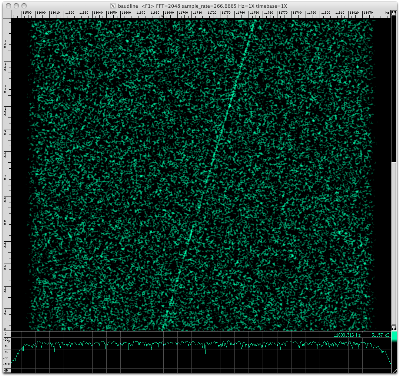The Voyager telemetry signal is Doppler drifting at a very linear -65.4 Hz / 139 seconds = -0.471 Hz/second rate due to the Earth's rotation. This manual drift rate measurement matches the Auto Drift rate value to within an error ±0.002 Hz/sec.

Conclusion
Baudline's Auto Drift feature made finding the drifting Voyager signal extremely easy. After it was found in the Average display the spectrogram display was zoomed and scrolled into position. A -0.47 Hz/sec drift rate seems a bit high. Need to check with the SETI Institute if this is a reasonable value. Next step demodulation ...

Data licensed through SETI.
Software licensed through SigBlips.

## Tuesday, November 09, 2010

### Carl Sagan's birthday pi

The NASA Kepler mission and the SETI Institute are celebrating Carl Sagan's birthday with an essay contest. I thought that I'd do something different and honor Carl Sagan by analyzing the digits of pi with the baudline signal analyzer. In Carl Sagan's novel "Contact" finding a signal hidden in the digits of pi was a theme element that was removed from the movie version. Since I've been spending a lot of my free time analyzing setiQuest data sets it seems rational to apply some of those same techniques to the irrational number pi.

So my plan is to use baudline and conduct Fourier analysis on the digits of pi in binary (base-2). Here is a description of the procedure:
1. Compute millions of decimal digits of pi.
2. Convert the decimal digits of pi to binary (base-2).
3. Analyze the binary digits with the baudline signal analyzer.

Existing software available on the Internet was used to calculate about 50 million decimal digits of pi. I wrote a simple O(n^2) base conversion routine that did multiple-digit base multiply and carry using integer division and modulo. For base-2 conversion accuracy verification I used the amazing Bailey–Borwein–Plouffe (BBP) formula algorithm to spot check several hexadecimal digits. The data was then feed into baudline using the standard input (stdin) or the raw parameter interface.

white uniform noise
The digits of pi are believed to be normal in that their distribution is random. Before looking at pi let us first take a look at the spectral characteristics of white uniform noise. Baudline settings:
1. Tone Generator set to output white uniform noise.
2. Input Devices tone generator loopback enabled.
3. Input Channel Mapping operation set to clip.
Here is a spectrogram of about one million samples of clipped uniform white noise:Here is an Average spectrum of 67 Msamples:pi binary (base-2)
The decimal (base-10) digits of pi were converted to binary (base-2). Here is a picture of the binary waveform:Here is a spectrogram of about one million samples of binary (base-2) pi:Here is an Average spectrum of 67 Msamples:Comparison
Let us compare the above uniform white noise and binary pi spectral displays. Click on the two spectrograms above for full size versions and see if you can find any significant differences. They look very similar to me. The two Average spectrums are fairly flat with equal energy and variance. Nothing stands out as odd, unusual, or different. So the conclusion from the perspective of these basic frequency domain tests is that white uniform noise is indistinguishable from the binary digits of pi.

If there is some hidden structure in pi then more sophisticated DSP techniques will need to be developed and utilized. Stay tuned ...

## Saturday, November 06, 2010

### setiQuest Lagrange-4

The baudline signal analyzer looked at the setiQuest Lagrange-4 data sets from 1420, 2008, and 3991 MHz. The Lagrangian points are locations in space that are in gravitational equilibrium. The L4 and L5 points are stable orbits which would make them an ideal place to store an "object" for a very long time. Another thought is that objects may tend to naturally collect at the L4 and L5 points. The Sun-Earth L4 point was the target of this setiQuest observation.

The following command line was used to stream the Lagrange-4 data files into a prototype version of baudline:

cat 2010-10-08-Lagrange-4_3991_3-8bit-* | ./baudline -session proto -stdin -format s8 -channels 2 -quadrature -samplerate 8738133 -pause -utc 0

1420 MHz
The frequency of interstellar Hydrogen. Here is the Average spectral display: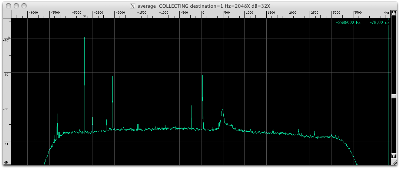Here is the signal at 1420 - 2.682582 = 1417.317418 MHz decimated by 4096. Note Hz=2X.This unusual signal jumps around making a drift measurement difficult. Four noise blobs of 33 Hz width that have some horizontal spectrum structure are present. The two middle blobs are about 100 seconds in duration and they appear to have different center frequencies.

Listen to this modulated signal in the following video. Select 720p HD and fullscreen for the best resolution.

Here is the signal at 1420 - 2.045546 = 1417.954454 MHz decimated by 4096. Note Hz=2X.This unusual wandering signal looks a lot like a 4X zoomed out version of the signal seen above at -2682582 Hz. The signal has a width of 8 Hz and drifts +19 Hz from the start to the end but it's motion looks more oscillatory.

Listen to this modulated signal in the following video. Select 720p HD and fullscreen for the best resolution.

Here is the chunk of bandwidth from 1420.029867 to 1420.032000 MHz.Three drifting signals are visible and each will be investigated below. Note that these three signals are about -460 kHz left of Hydrogen which has been a popular location for previous setiQuest signals of interest.

Here is the first signal at 1420 + 0.030525 = 1420.030525 MHz decimated by 4096. Note Hz=4X.A drifting random walk with a +107 Hz / 603 seconds = +0.177 Hz/sec drift rate. The lower half has an oscillatory drift shape with an 87 second period.

Here is the second signal at 1420 + 0.031453 = 1420.031453 MHz decimated by 4096. Note Hz=8X.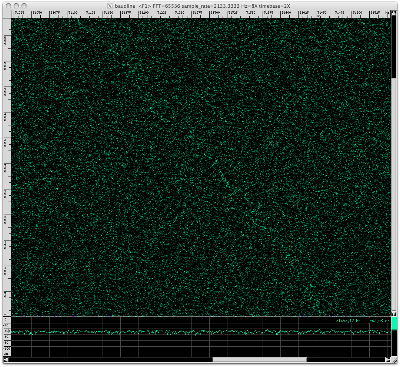This faint drifting random walk has a drift rate of +342 Hz / 603 seconds = +0.567 Hz/sec.

Here is the third signal that is at 1420 + 0.031681 = 1420.031681 MHz decimated by 4096.This wandering random walk is drifting at a net +17.1 Hz / 603 seconds = +0.0284 Hz/sec rate. Closer inspection by zooming into the time axis shows distinct frequencies that move by deltas of ±0.8 Hz. Using baudline's periodicity bar tool an extremely repetitive 3.753 symbol/second rate was measured. This looks very similar to the FSK-like zigzag modulation that was seen in the Kepler-4b redux analysis. That modulated signal was -483 kHz to the left of Hydrogen's corrected center of mass. This signal is about -460 kHz to the left of Hydrogen.

Listen to the 1420.031681 MHz modulated signal in the following video. Select 720p HD and fullscreen for the best resolution.

Here is the signal at 1420 + 0.036009 = 1420.036009 MHz decimated by 512.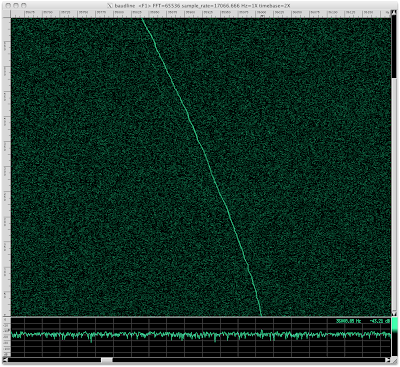This fairly constant non-stationary signal is drifting at +169 Hz / 603 seconds = +0.280 Hz/sec. This interesting thing about this about this signal is its slight wiggles and small discontinuous jumps in frequency. Since the decimation rate was only 512 these fluctuations are actually much greater when compared to the other spectrograms in this blog post.

Here is the chunk of bandwidth from 1420.029867 to 1420.032000 MHz.Again, three drifting signals are visible. We will zoom in to each signal to investigate further and measure its unique characteristics.

Here is the first signal at 1420 + 0.039448 = 1420.039448 MHz decimated by 4096. Note Hz=2X.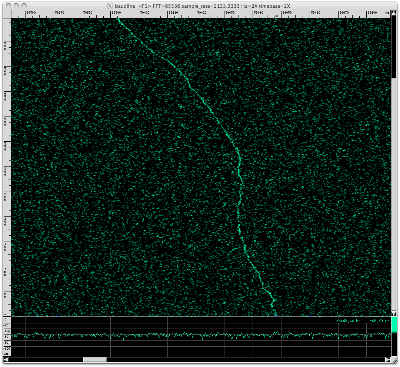Strange oscillating drift shape with a +56.0 Hz / 603 seconds = +0.0929 Hz/sec drift rate.

Here is the second signal at 1420 + 0.040146= 1420.040146 MHz decimated by 4096. Note Hz=4X.A drifting random walk whose drift has oscillatory as well as random elements. The net drift rate is +75.5 Hz / 603 seconds = +0.125 Hz/sec.

Here is the third signal at 1420 + 0.040632 = 1420.040632 MHz decimated by 4096. Note Hz=2X.An exact measurement is difficult but this weaker drifting random walk is moving at roughly -20.3 Hz / 462 seconds = -0.0439 Hz/sec.

Here is a signal at 1420 + 0.045422 = 1420.045422 MHz decimated by 4096.This narrow band noise signal is almost stationary with a -2 Hz / 603 seconds = -0.003 Hz/sec drift rate. Closer inspection of the lower third reveals alternating FSK-like blips with a 1.2 Hz delta and a repetitive periodicity of 7.1 seconds. The consistent spacing and periodicity suggest that this is not statistical noise.

Here is a signal which is -75 kHz left of Hydrogen peak at 1420 + 0.415107 = 1420.415107 MHz decimated by 4096. Note Hz=2X.Looks like scatter noise. Could be caused by multipath. Measuring drift rate is not possible.

Here is the very strong signal on the far right of the spectrum edge at 1420 + 4.315733 = 1424.315733 MHz decimated by 4096. Note Hz=4X.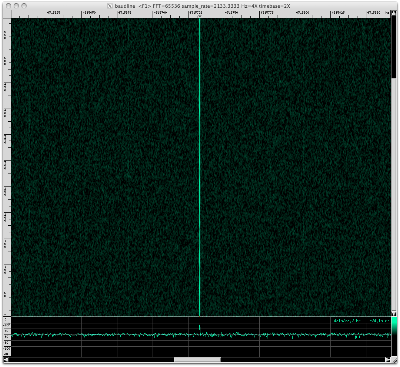This is a stationary pulsing tone. Several faint harmonic lines are visible on both sides of the main tone. Here is the Average display with the frequency axis zoomed out one notch.The harmonics are at ±50, ±73, ±120, and ±200 Hz which are all very suspicious values. Here is a spectrogram with the I&Q channels using the Histogram transform.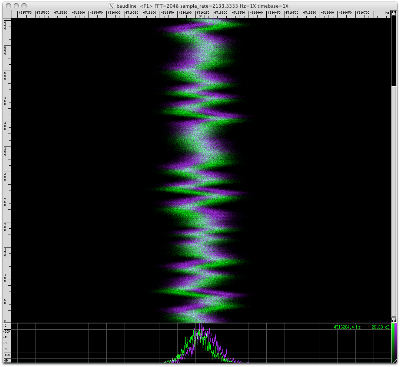The strong pulsing tone, ±50 and ±73 Hz harmonics, and wandering I&Q Histogram spectrogram were all seen in the Kepler-4b redux analysis with the -2422400 Hz signal. I suspect both were caused by the same distortion phenomena that is internal to the ATA. The Kepler-4b redux dataset was recorded almost 5 months prior.

2008 MHz
sqrt(2) * 1420 = 2008.This faint noise signal shifts -12 Hz in a fairly fast transition that last 101 seconds. At first this signal appears to be stationary but each section has a slight -0.0087 Hz/sec drift rate. A higher resolution signal is required to be certain but this has a classic Doppler flyby shape I often see with acoustic recordings of planes and helicopters.

Here is the signal at 2008 - 3.160508 = 2004.839492 MHz.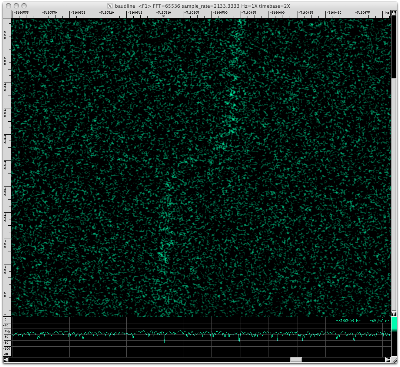This signal looks like the two-tone Doppler flyby above but it is +6400 Hz to the right in frequency and it has more well defined pulsing. Using baudline's periodicity bars a 26.3 second pulse rate was measured. The pulses before the flyby are also time aligned to those after.

Here is the signal at 2008 - 2.251751 = 2005.748249 MHz decimated by 4096. Note Hz=2X.This strange looking signal is about 2 Hz wide and it switches between two different linear drift rates. The net drift rate of -53 Hz / 579 seconds = -0.092 Hz/sec is composed of a slower -0.026 Hz/sec rate and a faster -0.21 Hz/sec rate. Baudline's periodicity bars show that the pulsing globs line up nicely with a 51 second periodicity spacing.

Here is the signal at 2008 - 2.184533 = 2005.815467 MHz.Stationary signal with zero drift rate. Repeated pulsing groups suggest signal could contain modulated content.

Here is the signal at 2008 - 2.177574 = 2005.822426 MHz decimated by 4096. Note Hz=2X.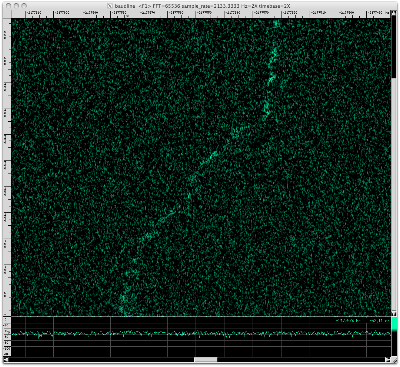This is a weaker version of the -2251751 Hz signal we saw above.

Here is the signal at 2008 - 2.103398 = 2005.896602 MHz decimated by 4096. Note Hz=2X.Another weaker version of the signal we saw above.

Here is the signal at 2008 - 1.948203 = 2006.051797 MHz decimated by 4096. Note Hz=8X.Narrow band noise-like pulses. Drift rate of -110 Hz / 271 seconds = -0.406 Hz/sec. The pulse bursts line up with a 87 second periodicity.

Here is the signal at 2008 + 1.094480 = 2009.094480 MHz decimated by 4096. Note Hz=8X.Drift rate of 240 Hz / 579 seconds = 0.415 Hz/sec. The previous signal above had a similar drift rate but looked completely different. Measured a periodicity of 52 seconds which matches that seen in the -2251751 Hz signal. That signal had the same periodicity but a quarter of the drift rate. It is interesting that this signal shares characteristics with two different signals, they are clearly related but not exactly.

3991 MHz
This frequency is expected to be a "bad band" filled with lots of C-band satellite signals.

This signal's bandwidth is 2.5 MHz wide. The spectrogram and Average spectrum look to be filled with an incredible number of signals. Let's zoom into the frequency axis of the Average spectrum:

There are thousands of narrow well defined signals everywhere you look in the spectrum Let's zoom in some more:

Using baudline's fundamental Hz measurement window the delta between peaks was accurately measured to be 1267.226 Hz. That is an interesting value because ...

Next let us decimate by 4096 and zoom into the strongest signal peak at 1502 kHz.

Slowly drifting to the left with a general drift rate of -0.91 Hz / 295 seconds = -0.0033 Hz/second. The signal erratically jumps between several discrete frequencies with deltas of {∆ 0.5, 1.0, 1.4, 3.2 Hz}. This signal is clearly modulated. Using the periodicity bars a symbol rate of 1 / 2.783 seconds = 0.359 symbols/second is measured.

Randomly spot checking 20 of the tone peaks reveals the same shape with varying amplitudes. So I believe that there are thousands of copies of this same signal. This could be caused by AM modulation, distortion in the ATA signal chain, or it could be a unique characteristic of this modulation scheme.

Listen to this modulated signal in the following video. Select 720p HD and fullscreen for the best resolution.

Something else extremely interesting is going on with this signal. Here is the autocorrelation spectrogram of the same signal seen above decimated by another factor of 64 for a total decimation ratio of 262144.The two patterns of interest are the strange shapes in the middle the 6+ holes near the bottom. They signify a more complex structure than you would expect from a drifting random walk. Similar shapes and holes were also seen in several other baudline-setiQuest analyses such as the two Kepler-4 blog posts.

Here is the spectrogram of the blip Fourier transform in phase space.Look along the vertical center-line (arrow) and notice the evenly spaced black holes near the bottom. Their periodic spacing measurement is 5.81 seconds for 7 consecutive major feature changes. This distribution is too uniform to be a statistic fluke. They signify 180º phase shifts which suggest a BPSK like modulation.

Here is a plot of the Autocorrelation transform using the Average window of the full bandwidth signal.The evenly spaced spikes represent that a repetitive pattern is present. This shape suggests the signal is direct-sequence spread spectrum (DSSS) and possibly CDMA. The spacing between autocorrelation peaks is 1 / 789.07 us = 1267.3 Hz which interestingly is within 0.1 Hz of the spectral measurement above.
The vertical dash-dot patterns represent changing bits (groups actually). Any common patterns you see are likely repeating header or idle sequences.

Conclusion
The Lagrange-4 datasets contained an incredible number of unique signals. Drifting random walks were a common theme in the 1420 MHz band while 2008 MHz was mostly populated with variations of a wider band Doppler flyby signal. Many of these signals had modulated features. Determining the source of these unknown signals is not really possible with the available information. This fairly sums up the challenge of SETI; detecting weak signals is easy, determining extraterrestrial origin is difficult.

The 3991 MHz band contained one 2.5 MHz wide signal that I suspect is CDMA. In the comments below a reader named Martin posted some extremely interesting information about the STEREO (Solar TErrestrial RElations Observatory) NASA satellites at the L4 and L5 positions (see plot below).

Martin mentions STEREO having a 633.245 bps data rate at 8.4 GHz. Twice this data rate is 1266.490 bps which is very close to the 1267.226 Hz spectral value and the 1 / 789.07 us = 1267.3 Hz autocorrelation rate I measured. The average error is 0.75 Hz which seems slightly greater than the accuracy level I felt baudline measured but since this signal is wiggling around by almost ±2 Hz it is in the realm of being a plausible match. Explaining how such a low baud rate signal gets down-converted by 4.4 GHz and expanded into a CDMA-like 2.5 MHz wideband signal is more difficult. In any event, this match is potentially an amazing discovery that should help in understanding the distortion characteristics of the ATA. Thank you Martin.

There were too many signals in the Lagrange-4 datasets to be able provide the quality of coverage each signal deserved. This blog post is the last time that I will attempt an exhaustive analysis of all the signals in a data set. It is a quantity vs. quality trade-off. Future baudline-setiQuest blog posts will focus on a single feature of interest. I also plan on incorporating more video clips so let me know what you think of them in the comments and how they might be more useful.